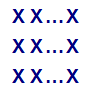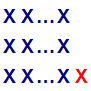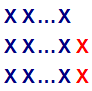#### You may also like### Calendar Capers

Choose any three by three square of dates on a calendar page...Make a set of numbers that use all the digits from 1 to 9, once and once only. Add them up. The result is divisible by 9. Add each of the digits in the new number. What is their sum? Now try some other possibilities for yourself!### Doodles

Draw a 'doodle' - a closed intersecting curve drawn without taking pencil from paper. What can you prove about the intersections?

# Take Three from Five

### Why do this problem?

This problem looks like a number task, possibly involving revision about multiples, but it becomes a question about establishing why something can never happen, and creating a convincing argument to show this. Students are used to considering the cases where numbers are either odd or even, and here they are being introduced to the idea that numbers can also be categorised into 1 more than, 2 more than, or exactly a multiple of 3. This provides an introduction to number theory and a possible springboard to the ideas of modulo arithmetic.

Algebraic proofs are often seen as the "gold standard" of mathematical rigour, but here is a nice example in which the visual proof can offer a deeper insight into the structure of the numbers modulo 3.

### Possible approach

It would be very useful for students to work on What Numbers Can We Make? before attempting this problem.

Introduce the problem in the way Charlie does in the video, by inviting students to suggest sets of five integers, circle three of them that add up to a multiple of three, and write down the total.

Don't say anything - let students work out what is special about the sum of the integers you select. Suggest that if they know what is going on they may like to choose five integers that stop you achieving your aim. At some stage check that they all know what is going on.

Challenge them to offer five integers that don't include three that add up to a multiple of $3$. Allow them time to work on the problem in pairs or small groups, and suggest that they write any sets they find up on the board. Students may enjoy spotting errors among the suggestions on the board.

Allow negative numbers, as long as they will allow you negative multiples of $3$ (and zero).

At some stage there may be mutterings that it's impossible. A possible response might be:
"Well if you think it's impossible, there must be a reason. If you can find a reason then we'll be sure."

Once they have had sufficient thinking time, bring the class together to share ideas.

If it hasn't emerged, share with students Charlie's representation from What Numbers Can We Make?

All numbers fall into one of these 3 categories:

Type A (multiple of $3$, i.e. of the form $3n$)Type B (of the form $3n+1$)Type C (of the form $3n+2$)We have found that trying to use algebraic expressions as above, is tricky - students often end up with n having two or more values at once.  For example, when considering the general sum of a type A, a type B and a type C number, students tend to write $3n+(3n+1)+(3n+2)$ without realising that this restricts them to the sum of three consecutive numbers.  Instead students need to realise that they should write something of the form $3n+(3m+1)+(3p+2)$.

Students are unlikely to know the notation of modular arithmetic, but the crosses notation above is sufficient for the context, and it suggests a geometrical image that students can use in explaining their ideas.

"Which combinations of A, B and C give a multiple of three?"
"Can you find examples in our list on the board where you gave me one of those combinations?"

A few minutes later...

"Great, then all you have to do is find a combination of As, Bs and Cs that doesn't include AAA, BBB, CCC or ABC!"

Later still...

"It's impossible! All the combinations will include either AAA, BBB, CCC or ABC!"

"OK, but can you prove it? Can you convince me that it's impossible?"

### Possible support

Start by asking students to select sets of three integers. You will always be able to choose two integers that add up to an even number. Challenge students to explain why.

### Possible extension

What Numbers Can We Make Now? is a suitable follow-up task.

A challenging extension:
You can always get a multiple of $2$ when you select $2$ integers from a set of $3$.
You can always get a multiple of $3$ when you select $3$ integers from a set of $5$.
Can you always get a multiple of $4$ when you select $4$ integers from a set of $7$?

What size set of integers do you need so that you can always get a multiple of $5$ when selecting $5$ of them?